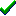# fg_3Dzcliptm()Win32DirectXFastgraph/Light

Prototype

int fg_3Dzcliptm (double *xyz_source, double *xyz_dest, void *uv_source, void *uv_dest, int n);

Function fg_3Dzcliptm (xyz_source() As Double, xyz_dest() As Double, uv_source() As Any, uv_dest() As Any, ByVal n As Long) As Long

function fg_3Dzcliptm (var xyz_source, xyz_dest : double; var uv_source, uv_dest; n : integer) : integer;

Description

The fg_3Dzcliptm() function clips a series of 3D (x,y,z) polygon vertices and corresponding 2D (u,v) texture map coordinates against the z plane clipping values defined by fg_3Dsetzclip(). Near clipping uses true clipping, but far clipping occurs only if the entire polygon lies beyond the far clipping plane. This function is called internally by Fastgraph's 3D functions and is not usually called directly by applications.

Parameters

xyz_source is the name of the array containing the 3D (x,y,z) vertices to clip. The first three elements of the array contain the coordinates for the first vertex, the next three elements are for the next vertex, and so on.

xyz_dest is the name of the array that receives the clipped 3D (x,y,z) vertices. The first three elements of the array will contain the coordinates for the first vertex, the next three elements will contain the next vertex, and so on. The xyz_dest array must be at least as large as the xyz_source array.

uv_source is the name of the array containing the (u,v) texture map coordinates for each (x,y,z) coordinate triple in xyz_source. The first two uv_source elements represent the (x,y,z) values at the first vertex in xyz_source, the next two uv_source elements are for the second vertex, and so forth. The fg_tmunits() function defines if uv_source contains integer or 32-bit floating point values.

uv_dest is the name of the array that receives the clipped 2D (u,v) coordinates. The first two elements of the uv_dest array will contain the coordinates for the first vertex, the next two elements will be for the next vertex, and so on. The fg_tmunits() function defines if uv_dest will receive integer or 32-bit floating point values. The uv_dest array must be at least as large as the uv_source array.

n is the number of vertices in the xyz_source and uv_source arrays.

Return value

The resulting number of vertices in the xyz_dest and uv_dest arrays.

Restrictions

Texture clipping is not supported for right-handed 3D coordinate systems.

See also

Contents
Fastgraph Home Page

copyright 2001 Ted Gruber Software, Inc.